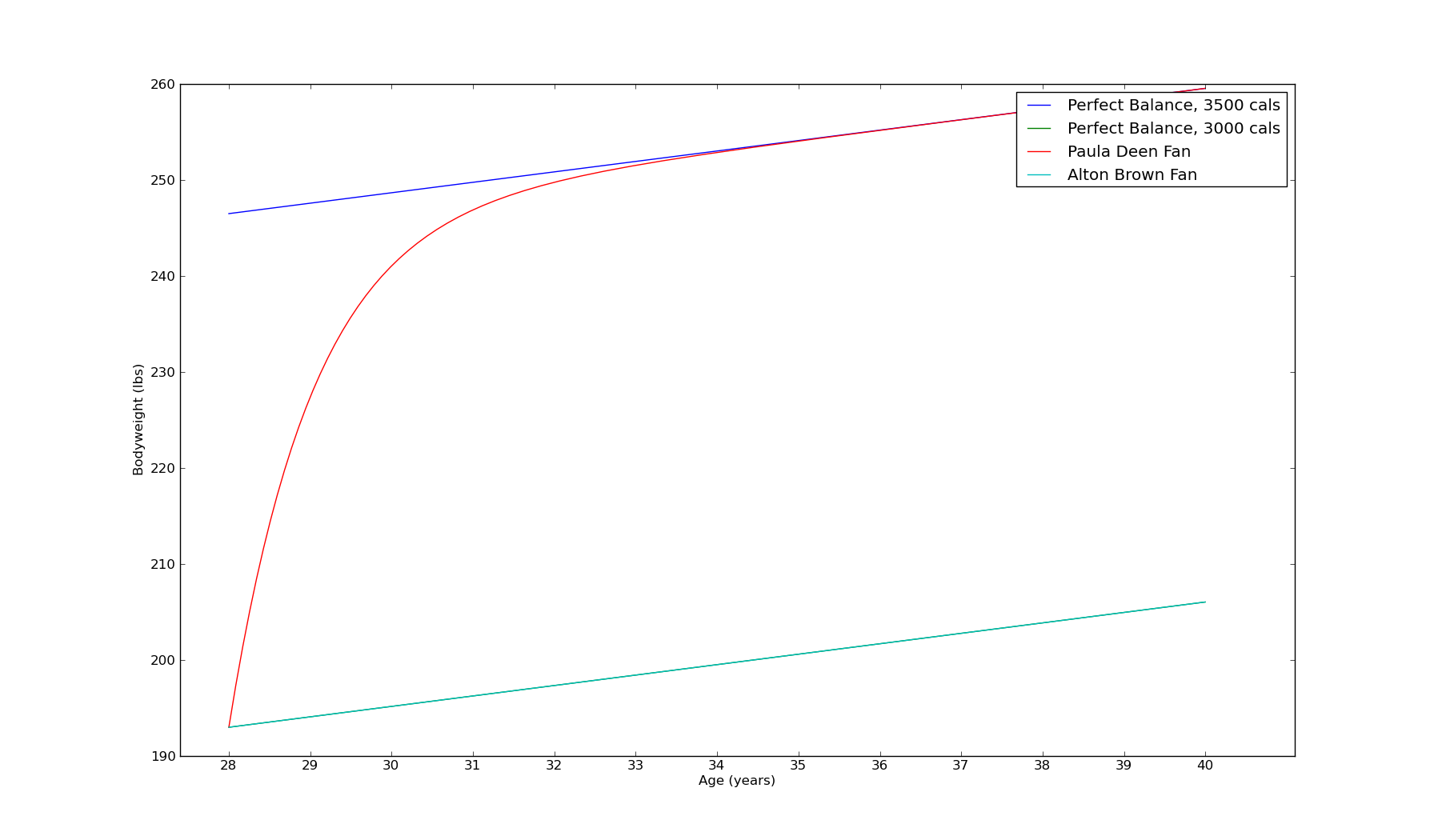A fairly recent trend in discussions of obesity is to focus on weight stability. Weight stability is a phenomenon by which a human maintains roughly the same bodyweight over a long period of time. Karl Smith recently brought it up, for example:

...its hard to square [theories of obesity based on lack of self control] with the stability of weight, which is probably the biggest or second biggest next to heritability, stylized fact about obesity. That is, if you always over eat you don’t get fat, you get fatter. Presumably, you will get fatter and fatter over time.

However, non-dieting obese folks have a weight stability that is roughly the same as thin people. Its as if they tried to go exactly to a particular too heavy weight and then stop and act like a thin person with 50 extra lbs.

Why does it stop like that?

That’s a puzzle.

It's a puzzle, but it's a puzzle with a solution. It turns out that the Calories In/Calories Out model of human metabolism completely explains the phenomenon of weight stability for the fat and thin alike.

The Calories In/Calories out model predicts that the human body converts calories to bodyfat at a rate of 3500 calories/1 lb of bodyfat::

``````Change in bodyweight = (Calories in - Calories out) x (1lb bodyfat / 3500 Cal)
``````

Calories in is simple, but calories out is a little tricky. The calories out portion is described by the Harris-Benedict equation. The Harris-Benedict equation (which I'll abbreviate to HBE) states that a man has a Base Metabolic Rate of

``````BMR = 66 + ( 6.23 x weight in pounds ) + ( 12.7 x height in inches ) – ( 6.76 x age in years )
``````

(the numbers are a bit different for women, but the equation is similar) and that

``````Calories out = E x BMR
``````

where E is a constant that varies with an individual's exercise rate. For a sedentary individual, E = 1.2, whereas serious athletes might have A as high as 1.9.

The key fact to note about this equation is that as a person's weight increases, his metabolic rate increases. This means that a fat person must eat more calories to maintain bodyweight than a thin person.

Now for some math. If you are unfamiliar with ordinary differential equations, you might want to skip to the pictures.

## The mathematical part

Based on this, we can derive an equation for a human's bodyweight as a function of time (with t having units of days):

``````w'(t) = c(t) - a - b w(t) + g t
``````

where

``````c(t) = Calories consumption as a function of time / 3500

a = E x (66 + 12.7 x height) / 3500

b = E x 6.23 / 3500

g = E x 6.775 / (365 x 3500)
``````

Here, `w'(t)` denotes the derivative of `w(t)` with respect to time. Now, suppose an individual consumes food at a rate of `c(t) = x + yt`. In that case, their bodyweight obeys the equation:

``````w'(t) = (x - a) - b w(t) + (g+y) t
``````

This equation has a simple solution:

``````w(t) = [(x-a)+bgt-g]/b^2 +  Const x exp(-bt)
``````

This is the equation of exponential decay - specifically, it shows that if a human eats a constant amount of food, their bodyweight will exponentially approach an asymptote which depends on `x` (their rate of consumption) as well as their age and activity level (which affect `a` and `b`).

## Results

I've graphed the result for a 6' tall 25 year old male who consumes 3000 Cals/day. After 3 years, regardless of his initial weight, his terminal weight lies between 190lb and 193lbs.Now consider two hypothetical people, both 6' tall, 28 years old, and exactly 193lbs. The first person becomes a fan of Alton Brown and cooks lots of healthy food. He continues eating 3000 Cals/day. He'll gain a few pounds before he reaches 40, but nothing remarkable. His weight will remain stable.

Now consider a second person, who becomes a fan of Paula Deen. By following her delicious recipes, he adds 5 tablespoons of butter y'all to his diet (500 extra calories). Over the next 3-4 years he gains 50lbs and then stops - all additional weight gain is due solely to aging, and is mirrored by the Alton Brown fan.The puzzle of weight stability is solved - fat people eat more than thin people, but by a constant amount. As they add food to their diet, their weight rapidly rises and then plataus at a higher level.

The difference between the 190lb man and the 250lb man is the latter eats everything the thin man eats, plus an extra 5 tablespoons of butter.

Here is the source code I used to create the graphs.